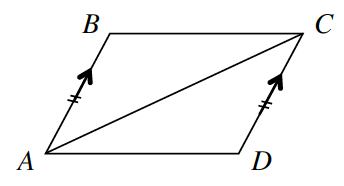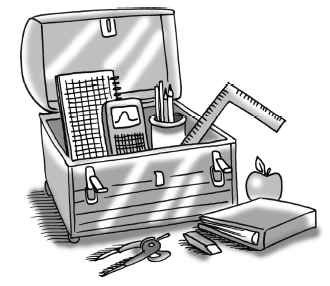Home > CCG > Chapter Ch7 > Lesson 7.2.5 > Problem7-100

7-100.

What else can you prove about parallelograms? Prove that if a pair of opposite sides of a quadrilateral are congruent and parallel, then the quadrilateral must be a parallelogram. For example, for the quadrilateral $ABCD$ at right, given that $\overline{AB}//\overline{CD}$ and $\overline{AB}\cong\overline{CD}$, show that $\overline{BC}//\overline{AD}$. Organize your reasoning in a flowchart. Then record your theorem in your Theorem Toolkit (Lesson 7.2.1A Resource Page).Be sure to supply the reasoning for each step.

If a pair of opposite sides is parallel and congruent then the quadrilateral is a parallelogram.
Use the if part of the statement as the givens, and the then part of the statement as the prove portion in the proof.

This flowchart has 8 bubbles. Bubble # 1 has an arrow, pointing down to bubble # 4. Bubble numbers 2, 3, and 4 have arrows, pointing down to bubble # 5. Bubble #5 has an arrow, pointing down to bubble #6. Bubble # 6 has an arrow, pointing down to bubble # 7. Bubble 7 has an arrow, pointing down to bubble # 8.

 Bubble # 1    $\begin{array}{l} \enclose{circle}[mathcolor=red]{\; \\ \quad \color{black}{\overline{AB} \parallel \overline{CD}} \quad \\} \\ \qquad \qquad \quad \text{Given} \end{array}$ $\Huge \downarrow$ Bubble # 2$\begin{array}{l} \enclose{circle}[mathcolor=red]{\; \\ \quad \color{black}{\overline{AB} \cong \overline{CD}} \quad \\} \\ \qquad \qquad \quad \text{Given} \end{array}$ Bubble # 3    $\begin{array}{l} \enclose{circle}[mathcolor=red]{\; \\ \quad \color{black}{\overline{AC} \cong \overline{CA}} \quad \\} \\ \enclose{}\qquad \qquad \quad \text{Segment} \cong \\ \qquad \qquad \quad \text{to itself} \end{array}$ Bubble # 4$\begin{array}{l} \enclose{circle}[mathcolor=red]{\; \\ \quad \color{black}{\angle BAC \cong \angle DCA} \quad \\} \\ \qquad \qquad \qquad \quad \text{Lines parallel} \; \rightarrow \\ \qquad \qquad \qquad \quad \text{alt. int. angles} \; \cong \end{array}$ $\Huge \searrow$ $\Huge \downarrow$ $\Huge \swarrow$ Bubble # 5$\begin{array}{l} \enclose{circle}[mathcolor=red]{\; \\ \quad \color{black}{\Delta ABC \cong \Delta DCA} \quad \\} \\ \qquad \qquad \qquad \quad \text{SAS} \,\cong \end{array}$ $\Huge \downarrow$ Bubble # 6      $\begin{array}{l} \enclose{circle}[mathcolor=red]{\; \\ \quad \color{black}{\angle DAC \cong \angle BCA} \quad \\} \\ \qquad \qquad \qquad \quad \cong \Delta \text{s} \; \rightarrow \; \; \cong \text{parts} \end{array}$ $\Huge \downarrow$ Bubble # 7$\begin{array}{l} \enclose{circle}[mathcolor=red]{\; \\ \quad \color{black}{\overline{BC} \parallel \overline{AD}} \quad \\} \\ \qquad \qquad \quad \text{Alt. int. angles} \; \cong \; \rightarrow \\ \qquad \qquad \quad \text{lines are parallel} \end{array}$ $\Huge \downarrow$ Bubble # 8$\begin{array}{l} \enclose{circle}[mathcolor=red]{\; \\ \color{black}{\quad \; \; ABCD \; \text{is a} \\ \quad \text{parallelogram}} \quad \\} \\ \qquad \qquad \quad {\text{Definition (two pairs of} \\ \text{sides are parallel)}} \end{array}$